Question

A 116 kg seal at an amusement park slides from rest down a ramp into the pool below. The top of the ramp is 1.85 m higher than the surface of the water and the ramp is inclined at an angle of 26.5 âˆ˜ above the horizontal.

Part A If the seal reaches the water with a speed of 4.10 m/s, what is the work done by kinetic friction?

Part B What is the coefficient of kinetic friction between the seal and the ramp?

1.Euphemia

a)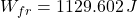, b)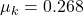Explanation:

The seal is modelled after the Principle of Energy Conservation and the Work-Energy Theorem: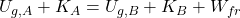a) The work done by the kinetic friction is: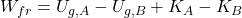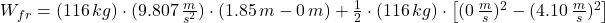b) The coefficient of kinetic friction is: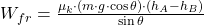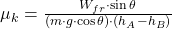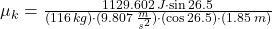2.thanhcong

(A) Work done by friction, Wf = 1128.1 J

(B) The coefficient of kinetic friction between the seal and the ramp,μ is 0.268

Explanation:

Given;

mass of seal, m = 116 kg

top of the ramp, h = 1.85 m

angle of inclination, θ = 26.5⁰

Part A

Apply the principle of conservation of Energy;

sum of initial potential energy and initial kinetic energy = sum of final potential energy, final kinetic energy and work lost due to friction.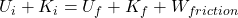Initial kinetic energy is zero, since the seal slides from rest.

Final potential energy is zero, at the end of the ramp, height is zero.

The equation above reduces to;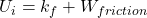Mgh = ¹/₂Mv² + Wf

Wf = Mgh – ¹/₂Mv²

Wf = (116 x 9.8 x 1.85) – (¹/₂ x 116 x 4.1²)

Wf = 2103.08 – 974.98 = 1128.1 J

Thus, work done by friction, Wf = 1128.1 J

Part B

work done by friction, Wf = Frictional force x distance moved by the seal down the slope

Wf  = Fk x d

Wf = μmgcosθ x d

d is the slope of the inclined ramp to the horizontal, this calculated using trigonometry ratio;

d (slope) = h/sinθ

d = 1.85/sin26.5

d = 4.146 m

Wf = μmgcosθ x d

1128.1 = μ x 116 x 9.8 x cos(26.5) x 4.146

1128.1  = μ x 4217.98

μ = 1128.1 / 4217.98

μ = 0.268

Thus, the coefficient of kinetic friction between the seal and the ramp is 0.268